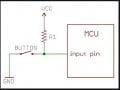# Logic circuit output for TC4071BP

#### Lemon_Foam

Joined Sep 18, 2017
39
I had built logic circuit as below:
When 3 sensor is on, it will give signal 1. Due to pull up resistor, the signal 1 will be reverse to 0. So, the signal input to the 2 logic gate will be 0. So, the signal Output A should be 0 too. But it always give me signal 1 at Output A when 3 sensor is turn on together

Is there any hidden concept behind this logic circuit? Since the output signal get was not expected.Last edited:

#### Lemon_Foam

Joined Sep 18, 2017
39
Here is your truth table -

View attachment 187471

Regards, Dana.
When 3 sensor is on, it will give signal 1. But due to the pull up resistor (R1,R2,R3), the sensor signal will become 0 before go into logic gate. So the expected output A result should be 0 right. But why it always give me a result of 1?

Joined Mar 10, 2018
4,057
A pullup normally implies a "1" unless its buss connection is pulled
down by an open drain / open collector sensor output. If sensor is not
plugged in a logic "1" will be present because of the pullup. If sensor
plugged in, then whatever the sensor output is passed into the gate.

Whats is the sensor ? Part number/description ?

Regards, Dana.

#### WBahn

Joined Mar 31, 2012
28,497
I had built logic circuit as below:
When 3 sensor is on, it will give signal 1. Due to pull up resistor, the signal 1 will be reverse to 0. So, the signal input to the 2 logic gate will be 0. So, the signal Output A should be 0 too. But it always give me signal 1 at Output A when 3 sensor is turn on together

Is there any hidden concept behind this logic circuit? Since the output signal get was not expected.

View attachment 187470
"Due to pull up resistor, the signal 1 will be reverse to 0. "

This doesn't make any sense on the surface of it.

How will a pullup resistor reverse a signal 1 to 0?

What are the voltage levels for your signals? Is a logic 0 a voltage of 0 V (or something close to it) and a logic 1 a voltage of 12 V (or something close to it)?

Your schematic shows an OR gate and an AND gate, but claims both are a TC4071BP, which is a quad two-input OR gate.

•absf

#### Lemon_Foam

Joined Sep 18, 2017
39
A pullup normally implies a "1" unless its buss connection is pulled
down by an open drain / open collector sensor output. If sensor is not
plugged in a logic "1" will be present because of the pullup. If sensor
plugged in, then whatever the sensor output is passed into the gate.

Whats is the sensor ? Part number/description ?

Regards, Dana.
I use the EE-SX951-W1M Omron Sensor (Light on). The sensor circuit will always turn on (always 12V to pass into logic gate----Logic gate=1).
When i cut the thrubeam between transmitter and receiver, the sensor circuit will turn off (No voltage goes into logic gate----Logic gate=0).

#### Lemon_Foam

Joined Sep 18, 2017
39
"Due to pull up resistor, the signal 1 will be reverse to 0. "

This doesn't make any sense on the surface of it.

How will a pullup resistor reverse a signal 1 to 0?

What are the voltage levels for your signals? Is a logic 0 a voltage of 0 V (or something close to it) and a logic 1 a voltage of 12 V (or something close to it)?

Your schematic shows an OR gate and an AND gate, but claims both are a TC4071BP, which is a quad two-input OR gate.
Logic 0 is 0v, Logic 1 is 12V. I attach the pull up resistor illustration diagram and assume button as sensor and MCU as logic gate. If Button close (sensor on), Vcc goes into ground. If button open(Sensor off), Vcc goes into gateLast edited:

Joined Mar 10, 2018
4,057

#### WBahn

Joined Mar 31, 2012
28,497
Logic 0 is 0v, Logic 1 is 12V. I attach the pull up resistor illustration diagram and assume button as sensor and MCU as logic gate. If Button close (sensor on), Vcc goes into ground. If button open(Sensor off), Vcc goes into gate

View attachment 187608
If the button is closed, Vcc does not go into ground -- but the input to the gate is pulled down to ground.

Are you declaring that the button being closed is the sensor somehow outputting a 1?

It sounds like if the sensor is ON, that the output of the sensor is LO (a logic zero), meaning that the sensor has an active-LO output. The pullup resistor is not somehow inverting the signal of the sensor, the output of the sensor is simply the opposite of the logical state of the sensor.